### Complete Data Visualization Guide: Python

“A picture is worth a thousand words”

-Fred R. Barnard

Data visualization is a visual (or graphic) representation of data to find useful insights (i.e. trends and patterns) in the data and making the process of data analysis easier and simpler.

Aim of the data visualization is to make a quick and clear understanding of data in the first glance and make it visually presentable to comprehend the information.

In Python, several comprehensive libraries are available for creating high quality, attractive, interactive, and informative statistical graphics (2D and 3D).

## Some popular data visualization libraries available in Python

• Matplotlib is one such popular visualization library available which allows us to create high-quality graphics with a range of graphs such as scatter plots, line charts, bar charts, histograms, and pie charts.
• Seaborn is another of Python’s data visualization library built on top of Matplotlib, which have a high-level interface with attractive designs. Moreover, it reduces the lines of code required to produce the same result as in Matplotlib.
• Pandas is another great library available in Python for data analysis (data manipulation, time-series analysis, integrating indexing of data, etc.). Pandas Visualization (built on top of Matplotlib) is a tool of Pandas library that allows us to create a visual representation of data frames (data aligned in tabular form of columns and rows) and series (one-dimensional labeled array capable of holding data of any type) much quicker and easier way.
• Plotly library is used for creating interactive and multidimensional plots making the process of data analysis easier by providing a better visualization for the data.

With this article, we will be able to visualize the data in different forms by learning how to plot data in different Python libraries and understand where to use which one appropriately.

Note: We can use Google Colaboratory to avoid the process of installation of libraries. All the libraries can be used by simply importing them in the notebook.

## Understanding the basics of Maplotlib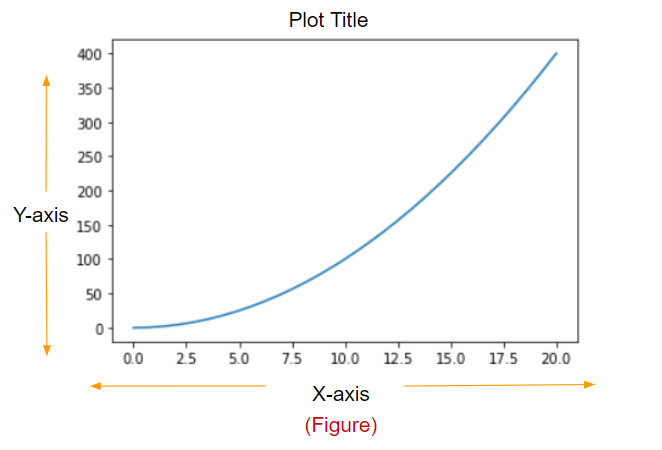(Image by Author) Elements of Graph
• Figure: The entire area where everything is being drawn. It can contain multiple plots with axes, legends, a range of axes, grid, plot-title, etc.
• Axes: The area under the figure where the plot is being constructed (or the area your plot appears in) is known as axes. There can be multiple axes in a single figure.
• Axis: This is the number line present in the graph which represents the range of values for the plot (X-axis and Y-axis as mentioned in the above figure). There can be more than two axis in the graph in the case of a multi-dimensional graph.
• Plot title: The title is positioned in the center above the axes, giving an overview of the plot.

## Importing the dataset

In this article, at various points, we will be using the Iris data set (as an example), which is free and is commonly used (since it is one of the best-known databases to be found in the pattern recognition literature).

We can import this data set in two ways:

### 1. Using Scikit-learn library: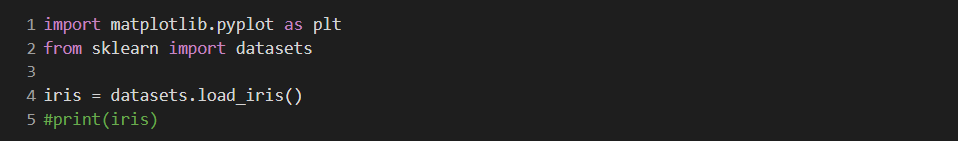### Without downloading the `.csv` file we can directly import the data set in the workspace using sci-kit learn library available in python.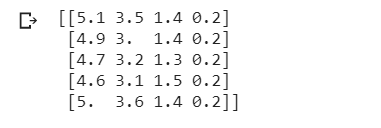(Image by Author) First five heads in the data set

### 2. Using Pandas library:

Using the above (by importing Pandas library) code, and downloading the `.csv` format of the dataset we can import the data in our workspace. These, are the first five elements in the `iris` dataset: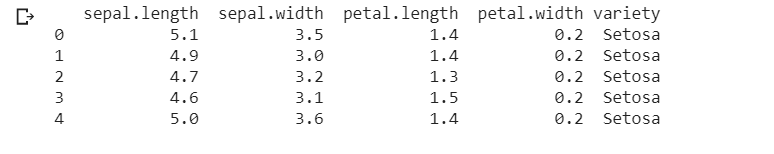(Image by Author) First five heads in the data set
Both of the above-mentioned methods can be used to import the dataset and to create graphs, but we will be using the latter because of the better readability of the data (as you can see the difference in the output results of both the methods).

## Getting started with Matplotlib

We begin by importing the library in our notebook by using the following code:
There are various styles available in this library for drawing the plot.

### Line plots

Line plot or line chart represents the data in a series (in continuation) showing the frequency of data along with the number line. It can be used to compare numerical sets of values. This is one of the most simple graphs that we can make using python.

Here, using the `numpy` `linspace()` function we will generate data-points and store them in variable `x` and calculate the square of values of `x` and store them in another variable `y` .

We will use `plt.plot()` function to plot the graph and `plt.show()` to display the graph.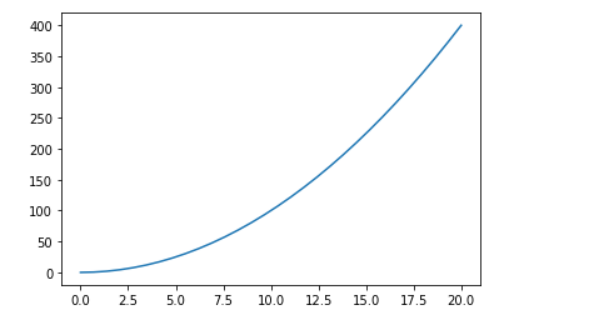(Image by Author) Line chart of y=x²

We can add some more functions to our plot to make it much easier to interpret.

• To add a label:`x-axis label` and `y-axis label` we will use `plt.xlabel()` and `plt.ylabel()` functions respectively.
• We can also give a title to our plot using the `plt.title()` function.
• A grid in the plot can simply be applied by calling `plt.grid(True)` function (makes data easier to interpret).

With the addition of these functions, the graph becomes much more readable and easier to analyze.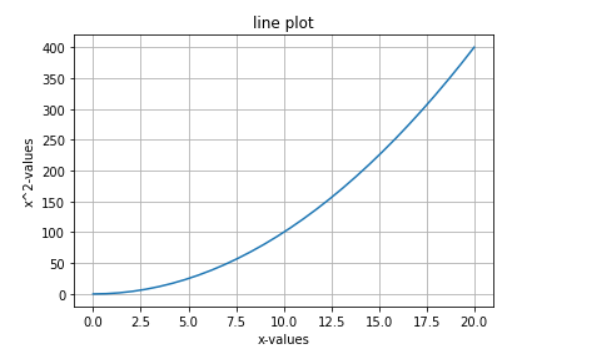(Image by Author) Line chart of y=x²

We can add more than one line to our plot and make them distinguishable by using different colors and some other features:

In the above code, we have added another variable `z=x**3` (z=x³) and changed the style and color of the line.

To change the color of a line in the line plot we have to add `color=''` parameter in `plt.plot()` function.

To change the style of a line in the line plot we have to add `linestyle=’’` parameter in `plt.plot()` function (or simply we can add ‘*’ or ‘- -’, etcetera).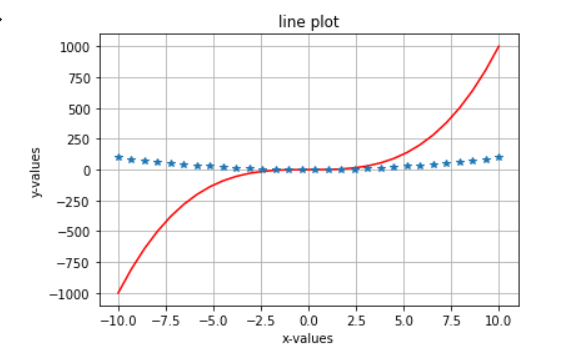(Image by Author) Line chart of y=x² and z=x³

This makes the extraction of information and comparison of data variables easier.

Similarly, we can create plots for mathematical functions as well:

Here, we have created a plot for `sin(x)` and `cos(x)` .

We can adjust the limit of axes by using the functions `plt.xlim(lower_limit,upper_limit)` for x-axis and `plt.lim(lower_limit,upper_limit)` for y-axis.

For further labeling of the plot, we can add `legend` with `plt.legend()` function, it will help to identify which line stands for which function.

### Subplots

For creating separate (multiple) plots in the same figure we can use the `plt.subplots(num_rows,num_cols)` function. Here the details of each subplot can be different.
`plt.sublots()` function creates a figure and grid of subplots, in which we can define the number of columns and rows by passing an `int` value as the parameter. Moreover, we can also change the spacing between the sublopts by using the `gridspec_kw={'hspace': , 'wspace': }` argument. After that, by simply using the index number for the subplot we can easily plot the graphs.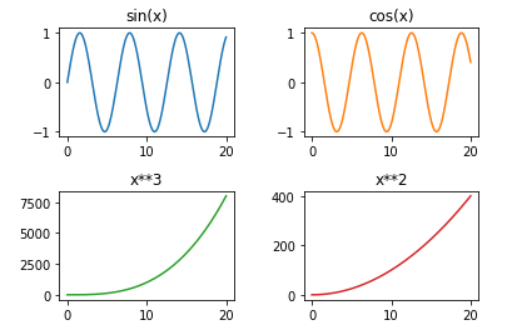(Image by Author) Four subplots in a single figure

### Scatter plots

This kind of plot uses ‘dots’ to represent the numerical data for different variables.

Scatter plots can be used to analyze how one variable affects the other variables. (We can use any number of variables we want to plot on the graph.)

We will use `dataset_name.plot()` function to create the graph and in parameters, we will apply the `kind = 'scatter’` with a label for `x-axis` and `y-axis` . Check out the example mentioned below (iris dataset).

Here, we are comparing the `petal length` and `petal width` of different species of flowers present in the dataset.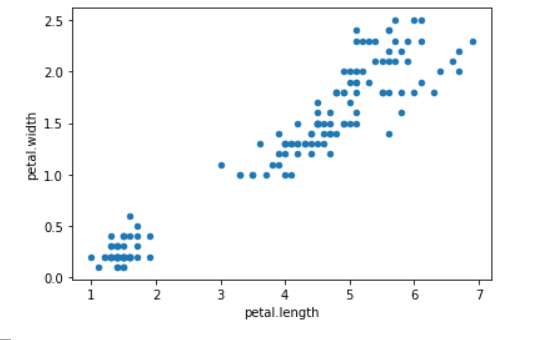(Image by Author) Iris dataset scatter plot

But, here it would be very difficult for us to analyze and extract information from this plot because we cannot differentiate between classes present.

So now, we will try another approach which will solve our problem. In this method, we will use `plt.scatter()` to create a scatter plot.

To change the color of dots based on the species of flower, we can create a dictionary with storing the colors corresponding to the names of the species. By using the `for` loop we create a single scatter plot of three different species (each represented by a different color).

This plot created is way better than the previous one. The data of species became easier to distinguish and gives an overall clarity for an easier analysis of information.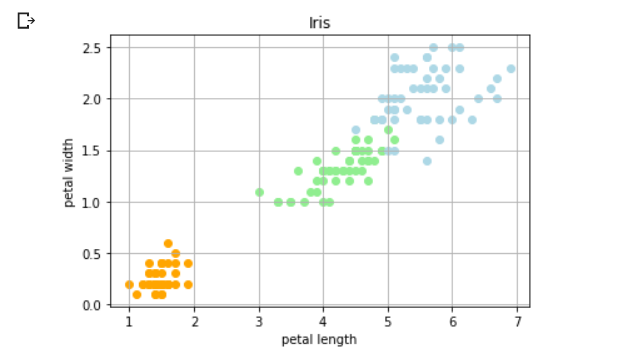(Image by Author) Iris dataset colored scatter plot

### Bar plots

Bar graphs can be used to compare categorical data. We have to provide the frequency and the categories, we want to represent on the plot.

Here we are using the iris dataset, to compare the count of different species of flowers (however, they are equal to fifty). To find the count of each unique category in the dataset we are using the `value_counts()` function. The variable `species` and `count` in the following code store the name of each unique category ( `.index` function) and the frequency of each category ( `.values` function)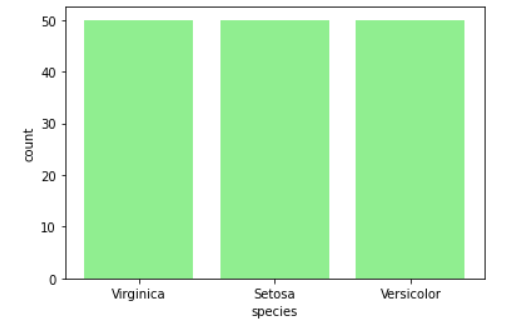(Image by Author) Count of different species of flowers in the iris dataset

This is the most basic kind of bar graph, you can try some variations of this plot like multiple bar plots in the same figure, change the width of bars (using `width=`parameter) or create a stacked bar plot (using `bottom `parameter).

### Box plots

Box plots help plot and compare the values by plotting the distribution of data based on the sample minimum, the lower quartile, the median, the upper quartile, and the sample maximum (known as the five-number summary). This can help us analyze the data to find the outliers and the variation in the data.

We have excluded the species column here since we are only comparing the `petal length, petal width, sepal length, sepal width` of all the flowers in the iris dataset. We create the box plot using the `.boxplot()` function.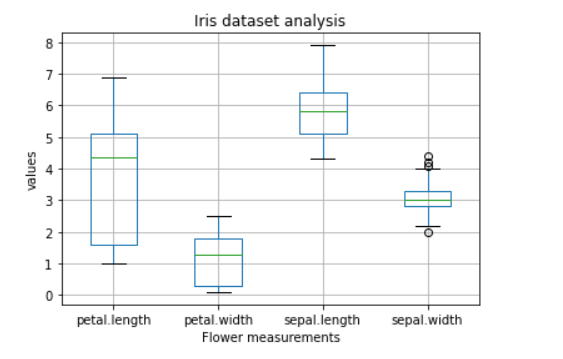(Image by Author) Box plot

### Histograms

Histograms are used for the representation of frequency distribution (or we can say probability distribution) of the data. We have to use the `plt.hist()` function to create the histogram plot and we can also define the `bins` for the plot (i.e. breaking down the entire range of values into a series of intervals and calculating the count of values falling in each interval).

Histograms are a special kind of bar graph.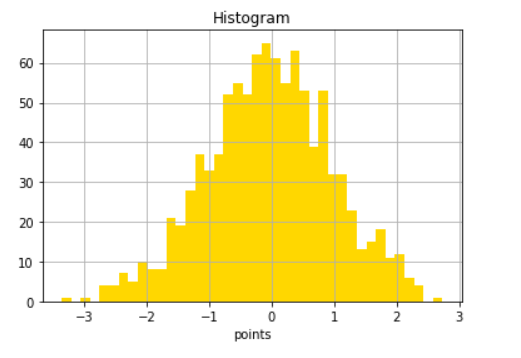(Image by Author) Histogram

### Error Bars

Error bar is an excellent tool to find out the statistical difference between the group of data by giving a visual representation of the variation in data. It helps to point the error and precision in the process of data analysis (and determine the quality of the model).

To plot the error bars, we have to use `errorbar()` function where `x` and `y` are data point locations, `yerr` and `xerr` define the size of the error bars (in this code we are only using `yerr`).

We can also change the style and color of the error bars by using `fmt` parameter (like we set the style to dots `’o’`in this particular example), `ecolor` for changing the color of dots and `color` parameter for changing the color of vertical lines.

By adding `loc = ''` parameter in the `plt.legend()` function we can determine the position of the legend in the plot.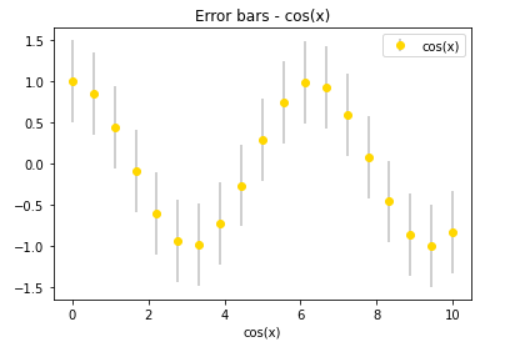(Image by Author) cos(x) error bar plot

### Heat maps

Heat maps are used to represent categorical data in the form of ‘color-coded image plot’ (values in the data are represented as colors) to find the correlation of the features in data (cluster analysis). With the help of heat maps, we can have a quick and deep analysis of the data visually.

In this example, we are using the iris dataset to create a heat map. `.corr() `is a panda’s data frame function used to find the correlation in the dataset. The Heat map is created by using the `.imshow() `function where we pass the `correlation` of dataset, `cmap` (for setting the style and color of the plot) as arguments. To add the colobar we use the `.figure.colorbar()` function. And finally to add annotations (the values you can see mentioned over the color blocks) we have used two for loops.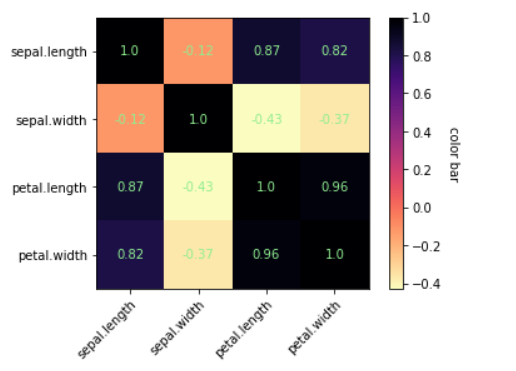(Image by Author) Iris dataset heatmap

### Pie charts

Pie charts are used to find the correlation (it can be percentage or proportion of data) between the composition of categories in the data where each slice represents a different category, giving the summary of whole data.
To plot the pie chart we have to use the `plt.pie()` function. To give a 3D effect to the plot we have used `shadow = True` parameter, `explode` parameter to show a category separately from the rest of the plot, and for displaying the percentage of each category we have to use `autopct` parameter. To make the circle proportionate we can use the `plt.axis('equal')` function.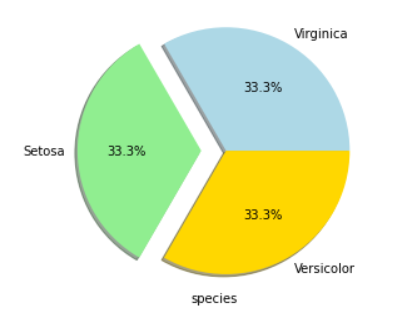(Image by Author) Pie chart matplotlib

## Seaborn

With the seaborn’s high-level interface and attractive designs, we can create amazing plots with better visualizations. Moreover, the lines of code required are reduced to a very great extent (as compared to matplotlib).

Code for importing the library in the workplace:

### Line plots

We can simply create the line plot in the seaborn library by using the `sns.lineplot()` function.

Here we can vary the color of grid/background using `.set_style()` function available in the library. And using `sns.lineplot()` function we can plot the line chart.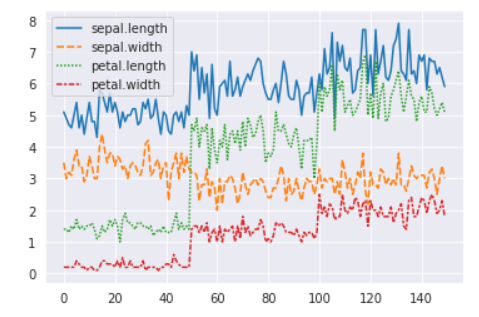(Image by Author) Line chart using seaborn library

### Scatter Plot

With the seaborn library, we can create the scatter plot in just a single line of code!

Here, we have used `FacetGrid()` function (with which we can quickly explore our dataset) to create the plot in which we can define `hue` (i.e. colors for scatter dots) and `.map` function to define the graph type. (Alternative method for creating a scatter plot is using `sns.scatterplot()` )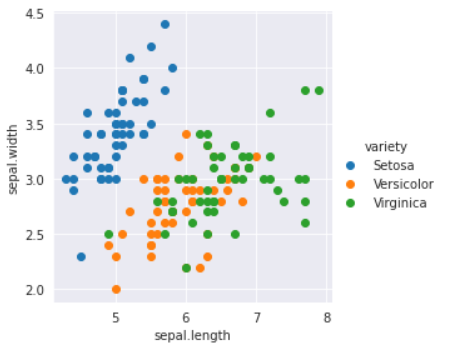(Image by Author) Scatter plot using seaborn library

### Bar plots

We can create a bar plot in the seaborn library by using `sns.barplot()` function.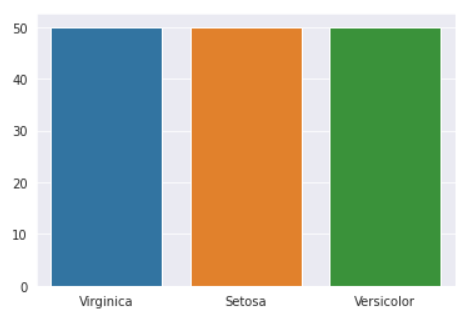(Image by Author) Bar plot using seaborn library

### Histogram

We can create a histogram in the seaborn library by using `sns.distplot()` function. We can also calculate probability distribution frequency (PDF), cumulative distribution frequency (CDF), and kernel density estimate (KDE) using this library for data analysis.

Seaborn gives some more features for data visualization than matplotlib.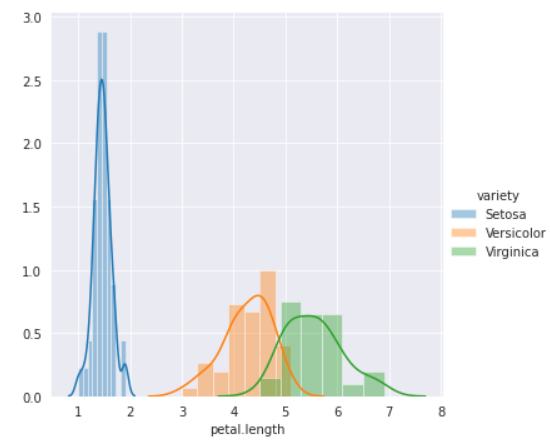(Image by Author) Histogram using seaborn library

### Heat maps

Seaborn is very efficient in creating heat maps by significantly reducing the lines of code to create the figure.

Multiple lines of code in matplotlib is reduced to just two lines!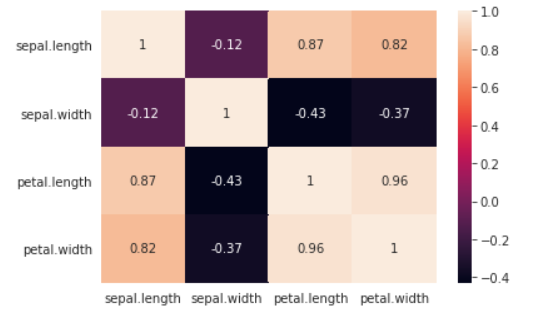(Image by Author) Heatmap using seaborn library

#### Pair plots

This is a unique kind of plot available in the seaborn library. This plots a pairwise relationship in datasets (in a single figure). This is an amazing tool for the purpose of data analysis.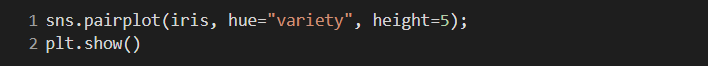By using `sns.pairplot()` function we can create pair plots ( `heigh``t`` `is used to adjust the height of the plots).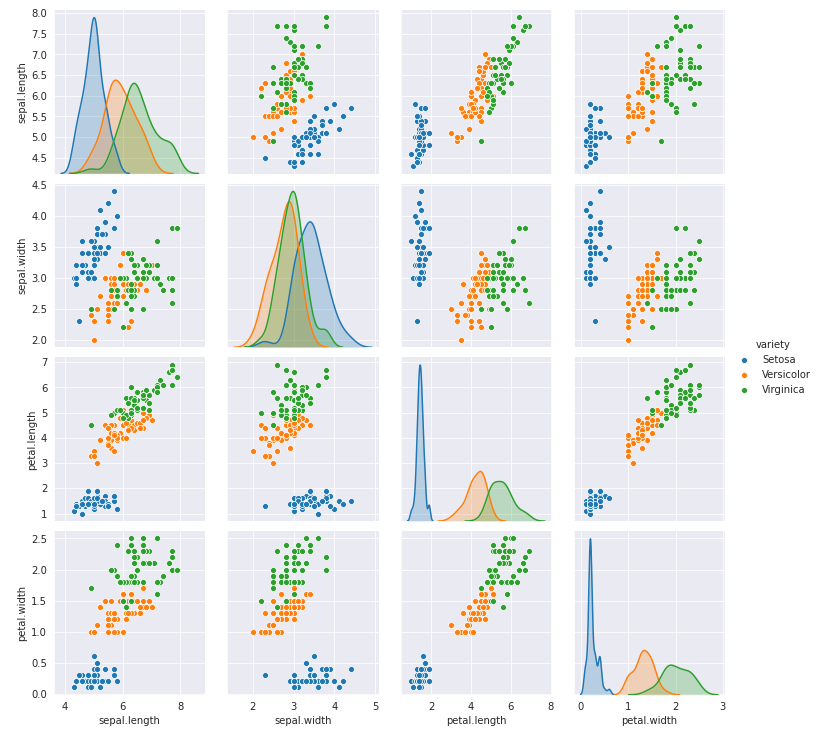(Image by Author) Pairplot using seaborn library

### Pandas Visualization

This library provides an easy way to plot graphs using pandas data frames and data structures. This library is also built on top of matplotlib thus requires fewer lines of code.

#### Histograms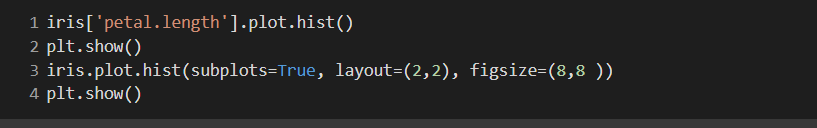It is very simple to create a histogram with this library, we simply have to use `.plot.hist()` function. We can also create subplots in the same figure by using `subplots=True` argument.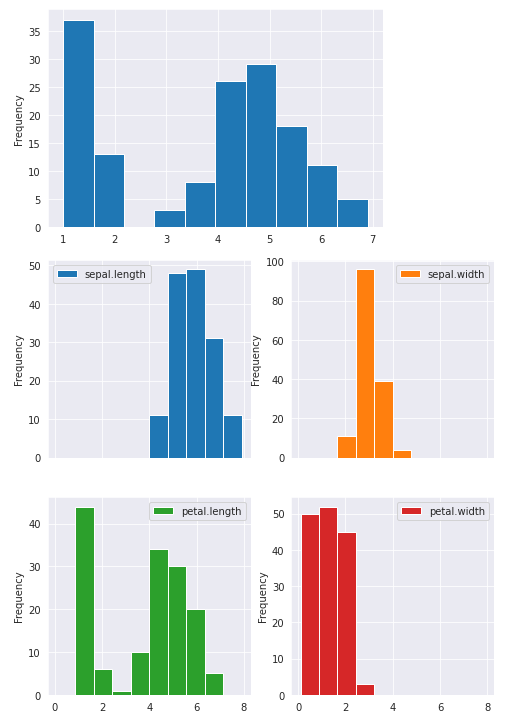(Image by Author) Histogram using the pandas library

### Line plots

We can create line plots using this library by using `.plot.line()` function. Legends are also automatically added in this library.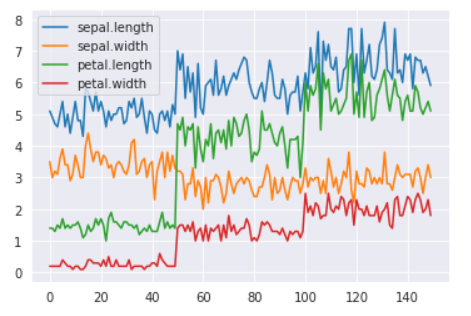(Image by Author) Line plots using the pandas library

## Plotly

With this library, we can create multidimensional interactive plots! This is easy to use library with a high-level interface. We can import this library by using the following code:

### 4D-plot (Iris dataset)

You try running this code on your own to check and interact with the plot.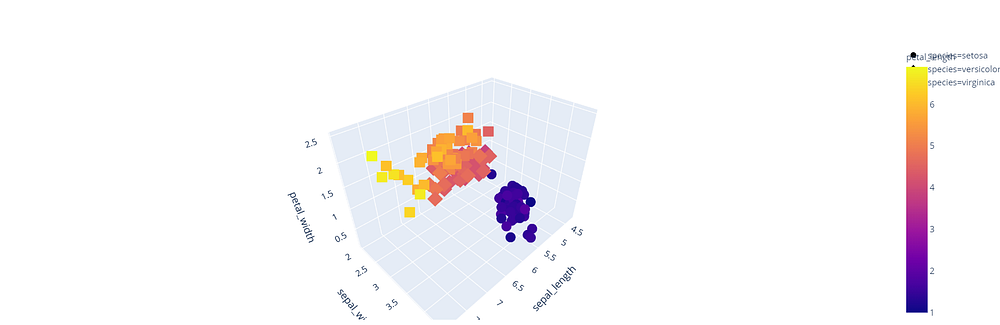(Image by Author) Interactive Multidimensional Plot

## Conclusion

I hope with this article you will be able to visualize the data using different libraries in python and start analyzing it.

For a better understanding of these concepts, I will recommend you try writing these codes on your once. Keep exploring, and I am sure you will discover new features along the way.

If you have any questions or comments, please post them in the comment section.

If you want to improve the way you code, check out our article:

https://patataeater.blogspot.com/2020/08/how-to-write-efficient-and-faster-code.html

`Resources:https://plotly.com/https://matplotlib.org/https://pandas.pydata.org/https://seaborn.pydata.org/`

1.Informative blog. Thank you for sharing with us..
Python Online Training

2.Hello,

Hirepython developer in us

Thanks & Regards
Tecocraft Ltd

3.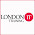Informative blog for python learner. Thanks for sharing.

python course london

4.Thanks for sharing all the information with us all.
Data Science Online Training
Salesforce Online Training

5.Thanks for Sharing This Article.It is very so much valuable content. I hope these Commenting lists will help to my website
angular js online training
best angular js online training
top angular js online training

6.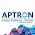Thanks for sharing this Information. Python Training in Gurgaon

7.This is a very nice one and gives in-depth information. I am really happy with the quality and presentation of the article. I’d really like to appreciate the efforts you get with writing this post. Thanks for sharing.
python training in pune

8.Very nice Article, good to see the response from the user and writer. I’d really like to appreciate the efforts you get with writing this post. Thanks for sharing. To know more: https://www.ethans.co.in/course/python-training-in-pune/

9.Good information you shared. keep posting.
data science certification

10.Good information you shared. keep posting.
artificial intelligence course in noida

11.Nice article. I liked very much. All the information given by you are really helpful for my research. keep on posting your views.
data analytics course in delhi

12.This comment has been removed by the author.

13.14."Very Nice Blog!!!

data science course in delhi ncr